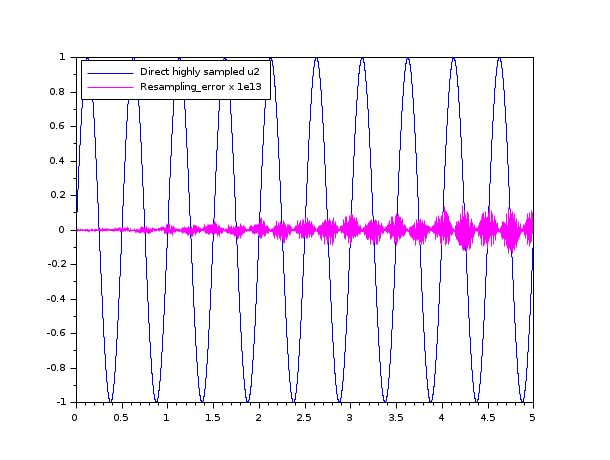Scilab Home page | Wiki | Bug tracker | Forge | Mailing list archives | ATOMS | File exchange
Change language to: English - Français - Português - 日本語 -
Справка Scilab >> Signal Processing > intdec

# intdec

Changes sampling rate of a signal

### Syntax

`[y]=intdec(x,lom)`

### Arguments

x

input sampled signal

lom

For a 1D signal this is a scalar which gives the rate change. For a 2D signal this is a 2-Vector of sampling rate changes `lom`=(col rate change,row rate change)

y

Output sampled signal

### Description

Changes the sampling rate of a 1D or 2D signal by the rates in lom

### Examples

```Fs1 = 1000;               // initial sampling frequency
Fs2 = 1400;               // targeted resampling frequency
t1 = linspace(0, 5-1/Fs1, Fs1*5)';
t2 = linspace(0, 5-1/Fs2, Fs2*5)';
F0 = 2;                    // Signal frequency
u1 = sin(2*%pi*F0*t1);
u2 = sin(2*%pi*F0*t2);     // Direct sampling at targeted frequency (as reference)
u2b = intdec(u1, Fs2/Fs1); // Resampled signal
clf
plot(t2,u2,"b", t2,(u2b-u2)*1e13, "m");
legend("Direct highly sampled u2","Resampling_error x 1e13","in_upper_left");```